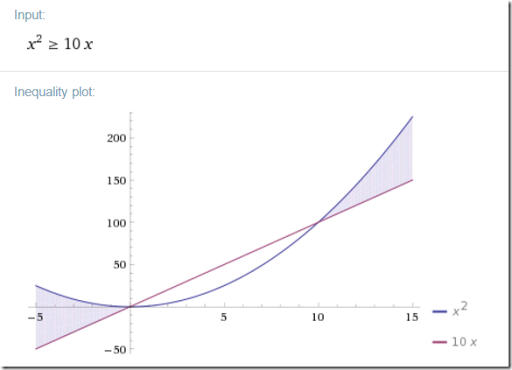## Wednesday, August 31, 2016

### Semi-continuous variables

A semi-continuous (or semicontinuous) variable $$x$$ is a continuous variable between bounds $$[\ell,u]$$ that also can assume the value zero. This is sometimes written as:
 $x \in \{0\} \cup [\ell,u]$

I also see sometimes $$x \in 0 \cup [\ell,u]$$. I am not sure which version is more correct. We assume $$0 \le \ell \le u$$ (negative bounds are not very well defined in conjunction with semi-continuous variables).

Semi-continuous variables are often used in situations where we don’t like to see very small values. A standard example is a portfolio optimization problem where many small allocations are not very attractive (e.g. because of a transaction costs). Instead we prefer fewer instruments in the portfolio with larger allocations. This situation can be handled using semi-continuous variables.

There are a number of ways we can implement this. Some useful, some not so much.

 \boxed{\begin{align}&\textbf{semi-continuous variable}\> x\\&x\in[\ell,u]\end{align}} Many MIP solvers have a built-in type for semi-continuous variables. The values $$\ell, u$$ need to be specified as bounds on $$x$$. (Don’t specify them in equations, as in this case $$x\ge\ell$$ has a different meaning than $$x^{lo}:=\ell$$). \boxed{\begin{align}&\ell\cdot \delta \le x \le u\cdot \delta\\&\delta \in \{0,1\}\\&x\in[0,u]\end{align}} This is a standard way to model semi-continuous behavior using an extra binary variable $$\delta$$. In most cases the “sandwich” equation needs to be implemented as two separate constraints. The bounds on $$x$$ are not so relevant (e.g. we could have used a free variable). \boxed{\begin{align}&x=\delta \cdot y\\&\delta\in \{0,1\}\\&y\in [\ell,u]\\&x\in [0,u]\end{align}} A simple, intuitive non-linear (quadratic) formulation. Unfortunately many MIQP/MIQCP solvers will not accept this construct. We see many interesting and rather scary error messages. My favorite: Return code - 5010 [MSK_RES_ERR_MIO_INTERNAL]: A fatal error occurred in the mixed integer optimizer. Please contact MOSEK support.(May be you are asked to contact the Mosek people to apologize for doing this). The reason for all this havoc: a nonlinear equality constraint makes the model non-convex. In some cases we can replace $$x=\delta\cdot y$$ by an inequality to make things digestible for convex solvers. \boxed{\begin{align}&x^2\ge \ell\cdot x\\&x\in [0,u]\end{align}} Very compact and looks harmless. So nice try, but unfortunately also non-convex. There is just no way to create a convex formulation without the aid of some form of binary (or at least discrete) variables.

Every post should have preferably some graph, so here we go. This plots $$x^2\ge \ell x$$ for $$\ell=10$$.